# Winding Numbers on Discrete Surfaces

ACM Transactions On Graphics (SIGGRAPH 2023)

Nicole Feng

Carnegie Mellon University

Mark Gillespie

Carnegie Mellon University

Keenan Crane

Carnegie Mellon University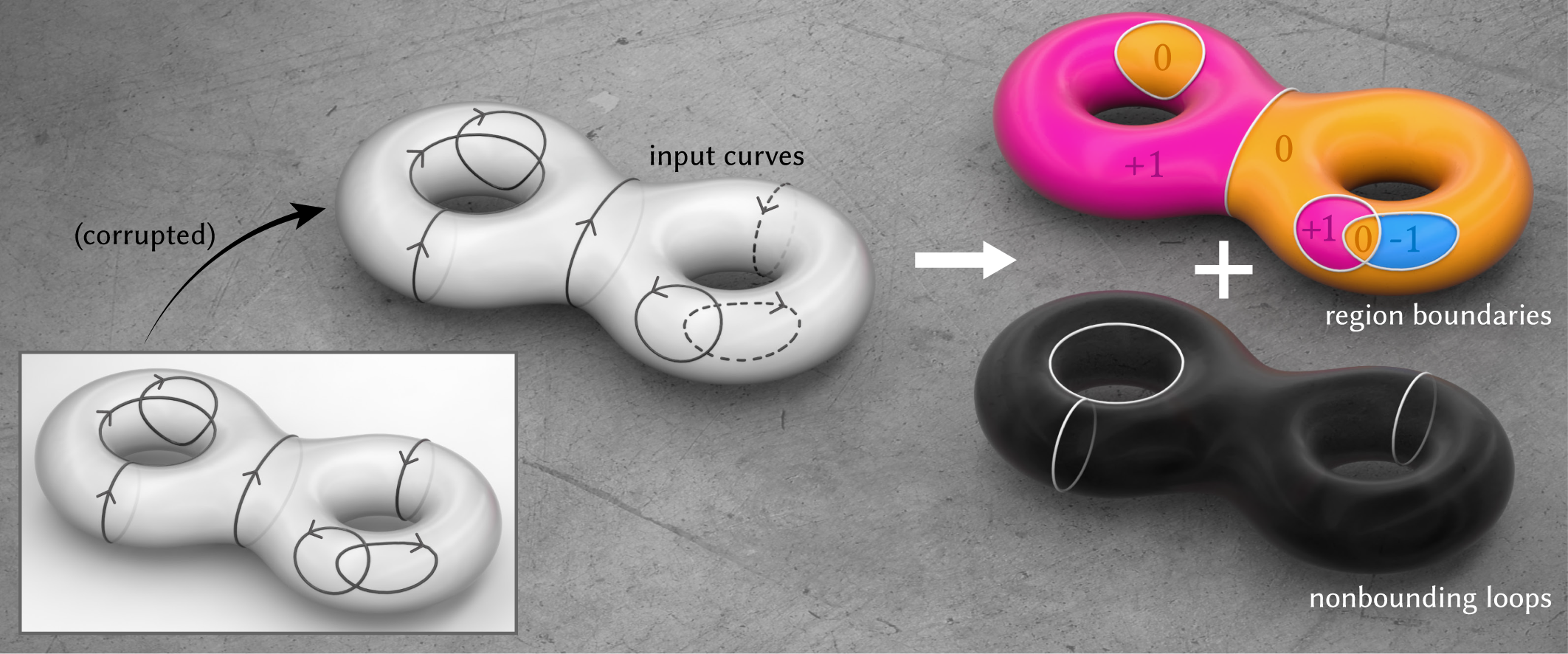# Abstract

In the plane, the winding number is the number of times a curve wraps around a given point. Winding numbers are a basic component of geometric algorithms such as point-in-polygon tests, and their generalization to data with noise or topological errors has proven valuable for geometry processing tasks ranging from surface reconstruction to mesh booleans. However, standard definitions do not immediately apply on surfaces, where not all curves bound regions. We develop a meaningful generalization, starting with the well-known relationship between winding numbers and harmonic functions. By processing the derivatives of such functions, we can robustly filter out components of the input that do not bound any region. Ultimately, our algorithm yields (i) a closed, completed version of the input curves, (ii) integer labels for regions that are meaningfully bounded by these curves, and (iii) the complementary curves that do not bound any region. The main computational cost is solving a standard Poisson equation, or for surfaces with nontrivial topology, a sparse linear program. We also introduce special basis functions to represent singularities that naturally occur at endpoints of open curves.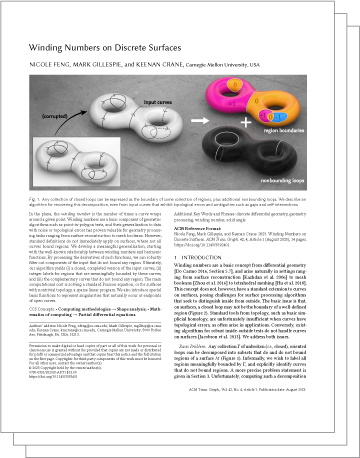Paper (4.4mb)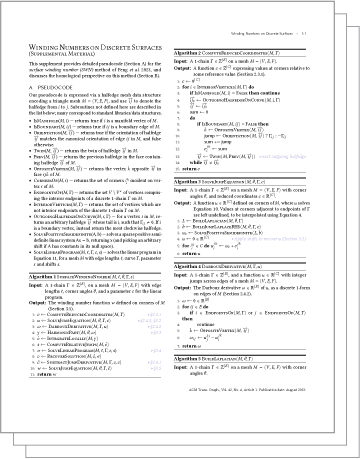Supplemental material (0.7mb)

Pseudocode & a discussion of homology.Perspectives on Winding Numbers (supplemental, 2.7mb)

A discussion of different mathematical and physical interpretations of winding number.C++ code

A C++ implementation of surface winding numbers (SWN).

Acknowledgements

This work was funded by an NSF CAREER Award (IIS 1943123), NSF Award IIS 2212290, a Packard Fellowship and gifts from Facebook Reality Labs, and Google, Inc.
@article{Feng:2023:WND, author = {Feng, Nicole and Gillespie, Mark and Crane, Keenan}, title = {Winding Numbers on Discrete Surfaces}, year = {2023}, issue_date = {August 2023}, publisher = {Association for Computing Machinery}, address = {New York, NY, USA}, volume = {42}, number = {4}, issn = {0730-0301}, url = {https://doi.org/10.1145/3592401}, doi = {10.1145/3592401}, journal = {ACM Trans. Graph.}, month = {jul}, articleno = {36}, numpages = {17} }

$$\newcommand{\assign}{\mathrel{#1}\mathrel{#2}}$$

# Errata

## Performance

The runtimes reported in Figure 17 in Section 5.2.2 are inaccurate (something went wrong with job scheduling.) The correct times are ~5x faster when measured on an Intel Xeon W-1250 CPU with 16 GB of RAM (in fact a less powerful processor than the one used in the original paper).## Reduced-size linear program (~100x speedup)

After publication, we realized a simple way to accelerate the linear program optimization in Section 3.4, which results in a ~100x speedup.

The original linear program (LP) simultaneously optimizes for both the sizes and locations of the residual function's jumps. Instead, we could first separately try to complete the curve Γ using a shortest-path heuristic (for example, Dijkstra), partitioning the domain M into several connected components.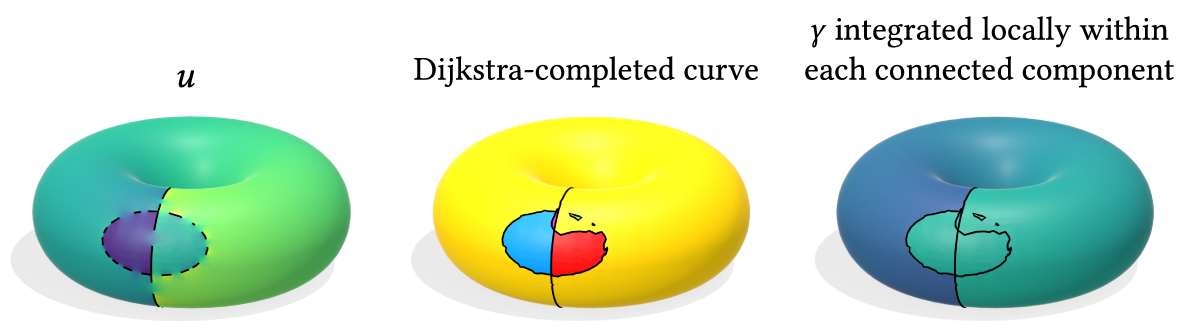We can locally integrate the harmonic 1-form γ within each connected component — as we did within each triangle face before — before using the original LP to minimize the L1 norm of jumps across connected components.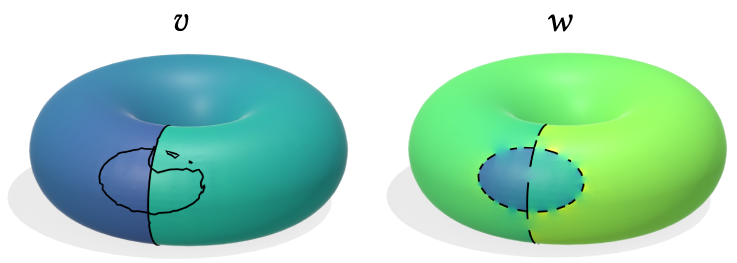The number of DOFs is reduced from the number of faces in the mesh to relatively few connected components.

Whereas the original LP took up to a few minutes in the worse cases, this approximate solution takes at most a few seconds, at the cost of a small decrease in accuracy on the benchmark.We could have also plugged in a different shortest-path method for the curve-completion step; for example, we could use weighted Dijkstra where the weights correspond to the magnitude of the gradient of the jump harmonic function $$u$$.

# Talk at SIGGRAPH 2023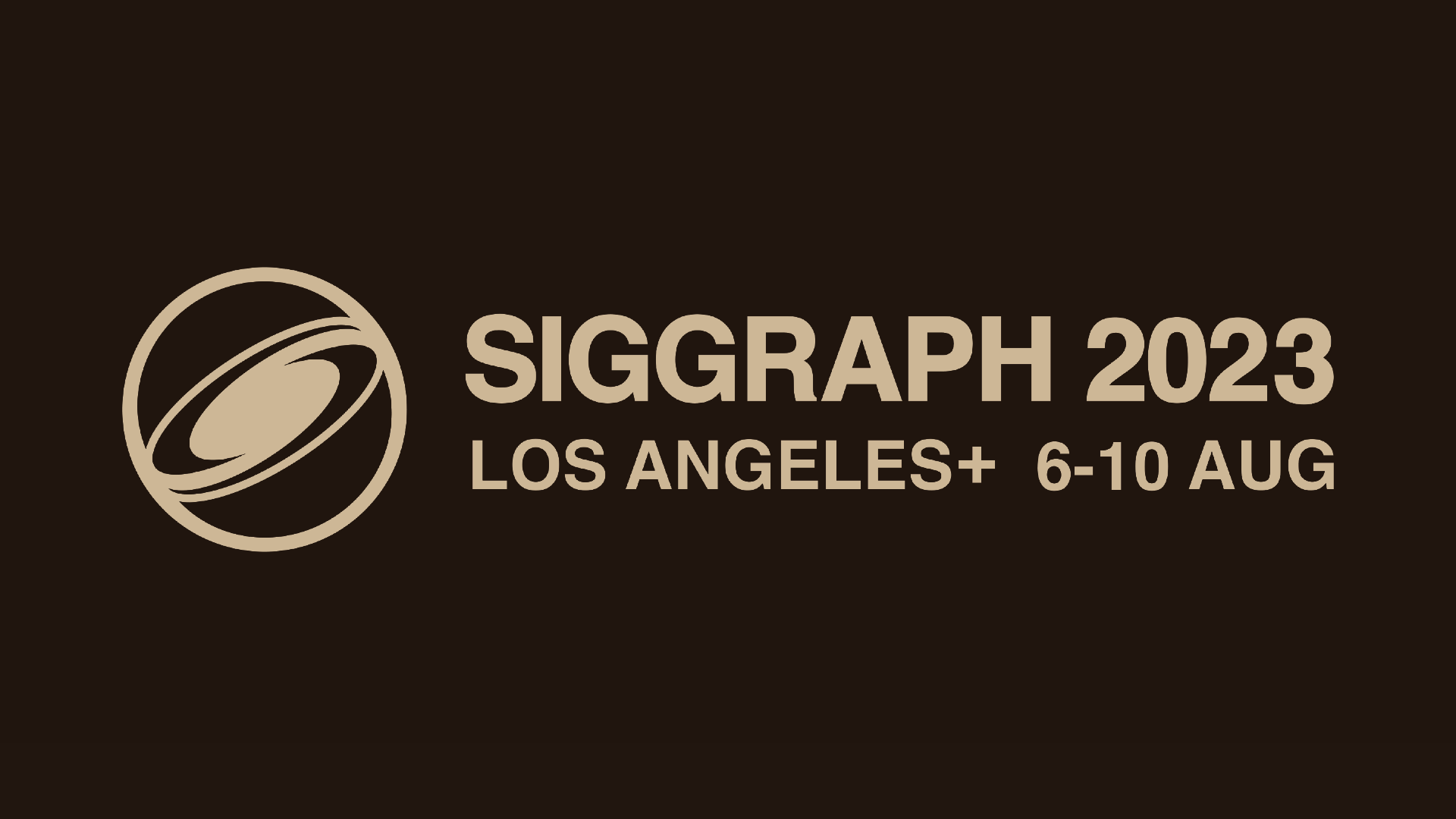Slides for 10-minute SIGGRAPH talk (15.8mb)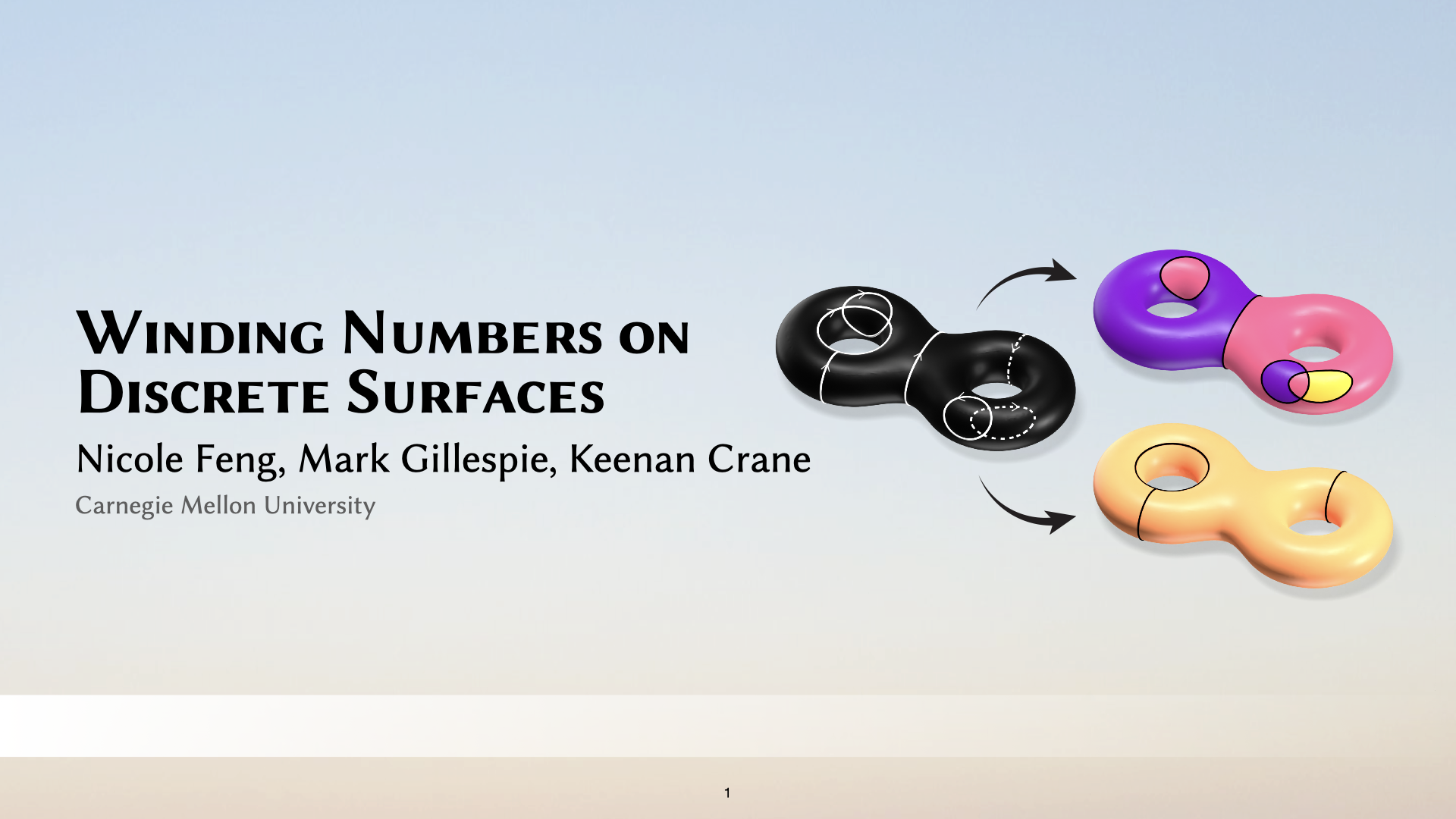Slides for 50-minute talk (45.2mb)

# What is this paper about?

For a given point on a surface, we would like to know whether it is inside or outside the given curve. For example, coloring points on this cow according to inside vs. outside would look like this: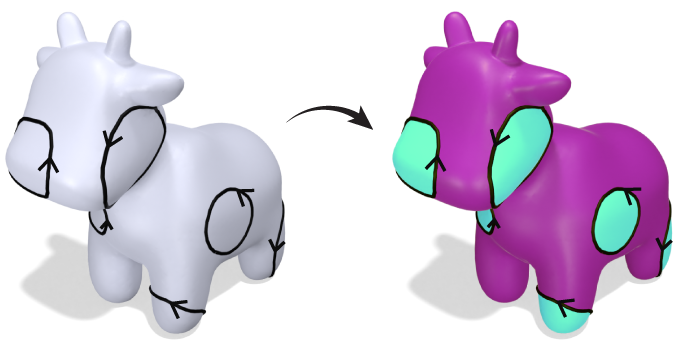But on surfaces, not all closed curves bound regions:And the solution is not so simple as classifying loops as "bounding" vs. "nonbounding", since nonbounding loops can combine to bound valid regions (figure reproduced from Riso et al. 2022, Fig. 4):More generally, we would like to answer "inside or outside?" on all types of surfaces, in addition to those that are multiply-connected. This question gets even harder to answer if some of the curves have been corrupted somehow and are now broken.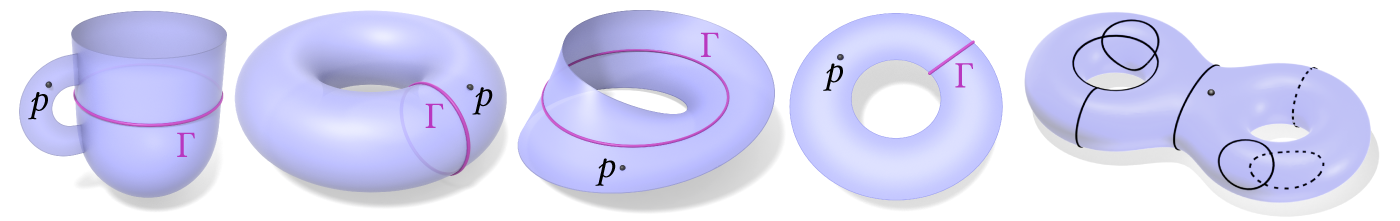Our starting point is a quantity called the winding number – also known as solid angle – which appears widely across math and physics. The winding number (solid angle) at a point $$p$$ is perhaps most commonly expressed as in an integral over the curve. Intuitively, we measure the infinitesimal angle subtended by the curve at $$p$$, and sum up all the angles over the curve. For a closed curve in the plane, this gives us a piecewise constant function whose value at each point tells us how much the curve winds around it.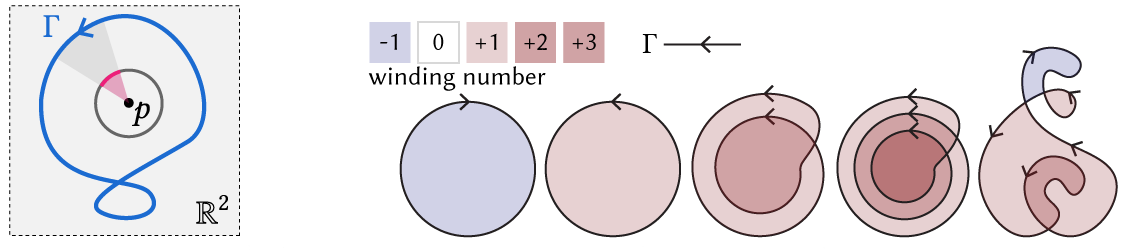Folks in graphics and geometry processing have already used solid angle to great success! Perhaps the most well known examples are the mathematically equivalent methods of Poisson Surface Reconstruction [Kazhdan et al. 2006] and generalized winding number [Jacobson et al. 2013]. However, all past methods are only applicable to Euclidean domains, not surfaces. For further discussion of why usual interpretations of solid angle don't apply in surface domains, see our supplemental, Perspectives on Winding Numbers.

There's been relatively little mathematical or algorithmic treatment of winding number on surfaces. Recently, Riso et al. 2022 developed the algorithm BoolSurf for computing booleans on surfaces – but assume that the input curves are closed, and already partitioned into loops. It remained an open question of how one could optimally infer curve topology from unstructured input. More generally, we aimed for a principled handling of surfaces with boundary, and other types of surfaces. Concretely, our algorithm does the following: Given an unorganized collection of oriented curves with no additional structure, we robustly determine inside vs. outside on general surfaces. In the process, we determine the bounding vs. nonbounding components of the curve, and produce a closed, completed version of the input curve.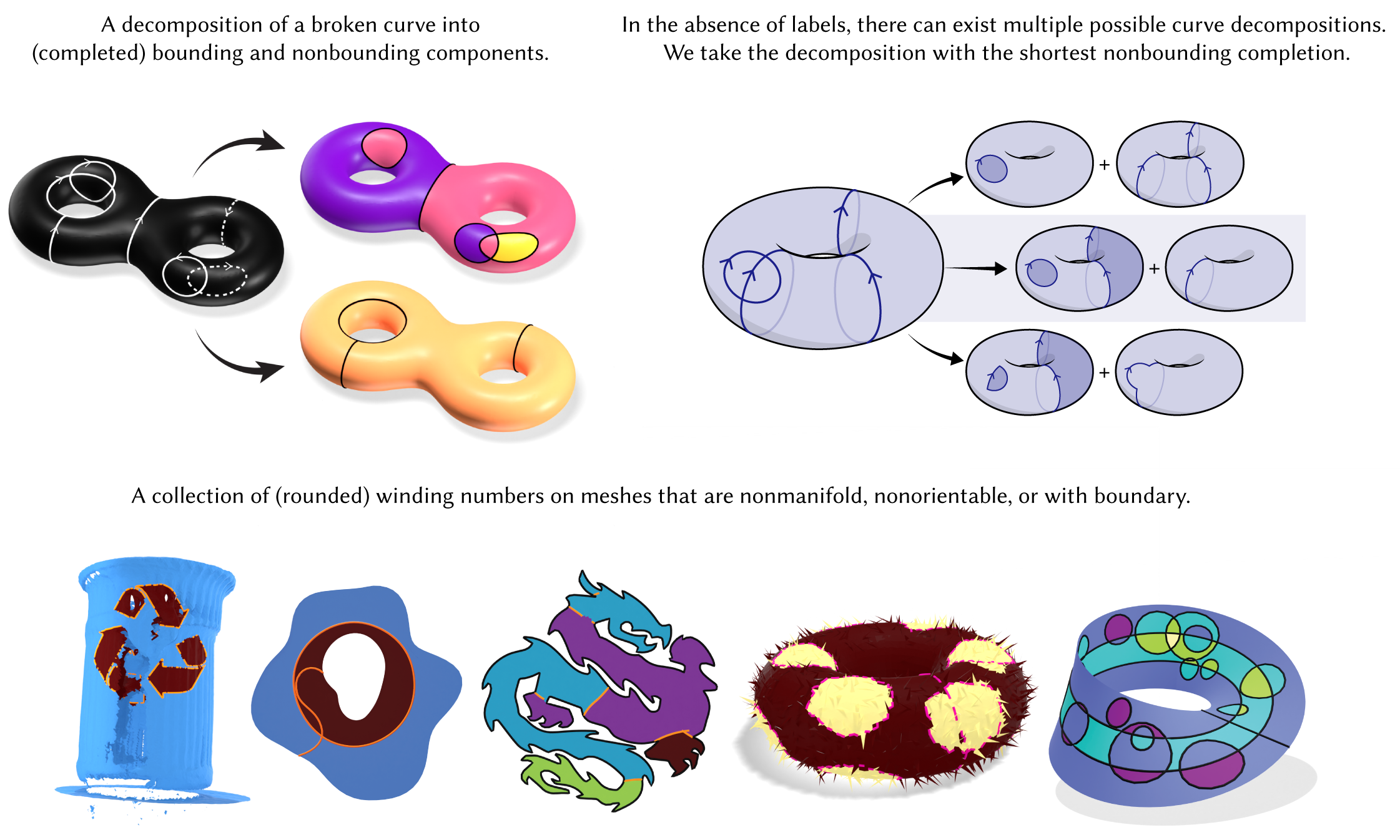First, we recognize that winding number/solid angle can be characterized as a jump harmonic function, meaning a function that is harmonic everywhere – including at points on the curve – modulo the jumps. (The function only fails to be harmonic at the curve's endpoints, where there is a branch point singularity.)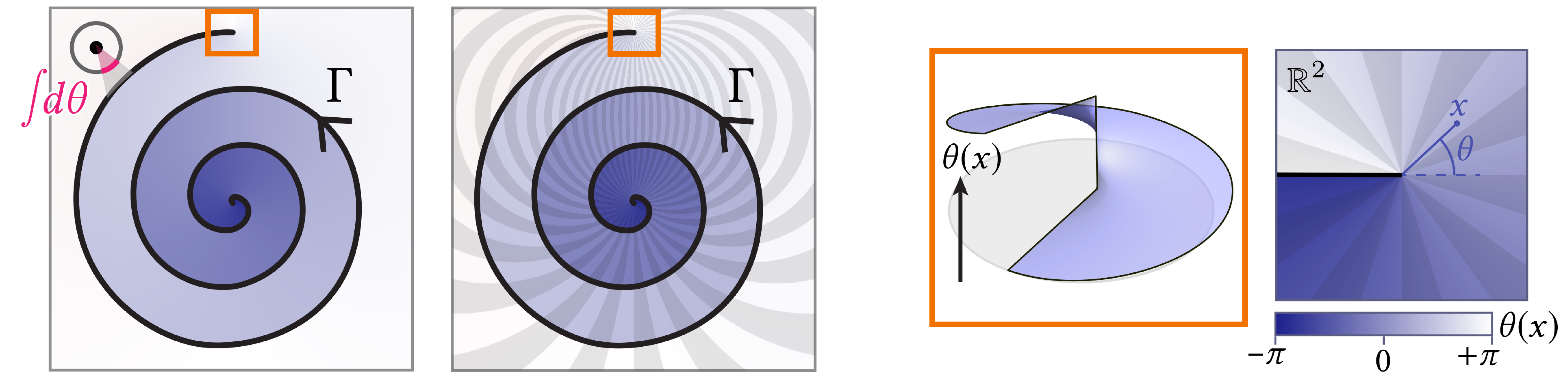Hence our starting point to winding numbers on surfaces is the jump Laplace equation,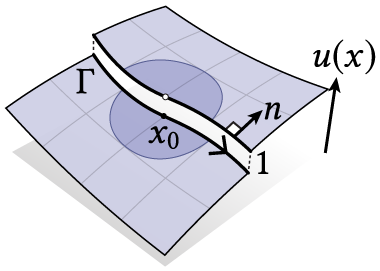\begin{align*} \Delta u &= 0 &\text{on } M\setminus\Gamma\\ u^+ - u^- &= 1^* &\text{on } \Gamma \\ \partial u^+/\partial n &= \partial u^-/\partial n &\text{on } \Gamma \end{align*}

*More generally, $$u^+(x) - u^-(x) =$$ number of times $$\Gamma$$ covers $$x$$.

Basically, the jump Laplace equation corresponds to the standard Laplace equation, plus a condition that stipulates the solution must jump by the specified amount across the curve $$\Gamma$$, and a compatibility condition that ensures the solution is indeed harmonic "up to jumps" everywhere on $$M\setminus\partial\Gamma$$.

The jump Laplace equation is essentially the PDE version of solid angle/generalized winding number. Simply solving the jump Laplace equation, however, doesn't yield a winding number function on surfaces, because of the influence of nonbounding loops. Formally, (non)bounding loops are loops that are (non-)nullhomologous: congruent to zero in the first homology group $$H_1(M): \rm{ker}(\partial_1)\setminus\rm{im}(\partial_2)$$ of the surface $$M$$. In words, nonbounding loops are closed curves which don't bound regions.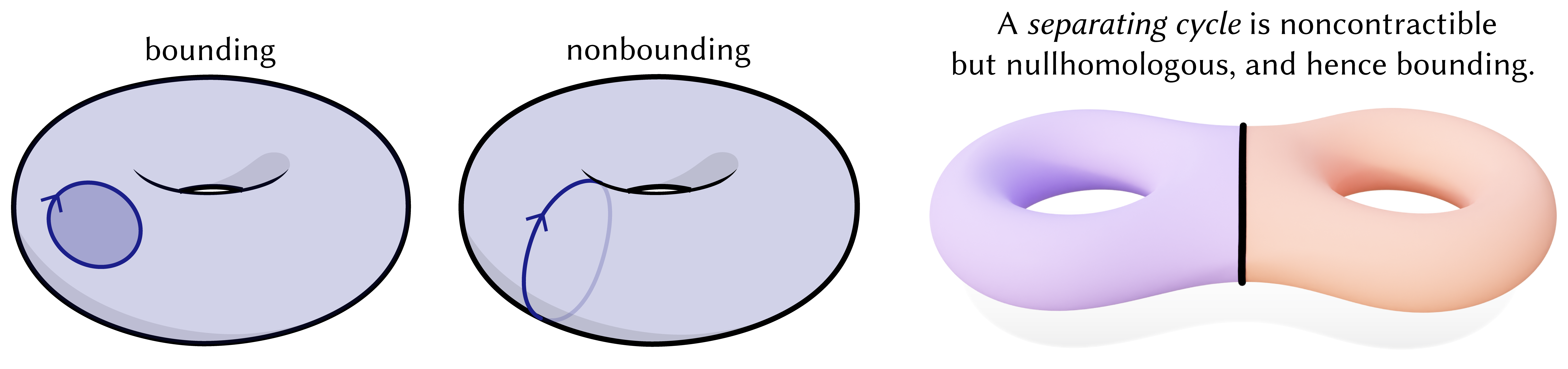Great, we've identified the problem! But homology only directly applies to closed curves. So we use de Rham cohomology to instead process functions dual to curves. We start by taking an appropriate derivative of our jump harmonic function. Once in the "gradient domain", our problem of decomposing a curve into its bounding and nonbounding components – meaning components that can and cannot be explained as region boundaries – becomes the problem of decomposing a vector field into components that can and cannot be explained by a potential, via Hodge decomposition.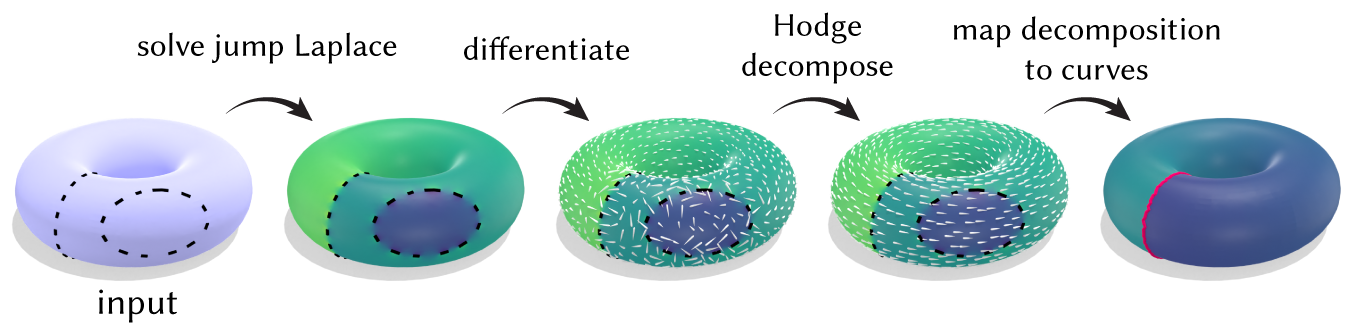Because we work with smooth functions defined globally on the domain, our algorithm is much more robust than if we had tried to work directly with sparse, singular curves. In all of this, jump harmonic functions play a key role in allowing us to translate between curves and differential 1-forms, since they encode both curves (where it jumps discontinuously) and its homology class (via its derivative.)Discretely, we represent curves and regions as chains on a triangulated surface. The resulting algorithm entails solving a sparse linear system (Laplace equation) with the usual cotan-Laplace operator. On surfaces of nontrivial topology, we solve another sparse linear system (another Poisson problem) for the Hodge decomposition, and a sparse linear system to map the decomposition of our 1-form to a decomposition of our curve.

# Discussion

## What if the curve isn't constrained to mesh edges?

Our algorithm assumes the input curve $$\Gamma$$ is restricted to mesh edges. Can we relax this assumption?

We can: Instead of a Laplace equation with jump conditions, we may consider an equivalent Poisson equation with a source term encoding the gradient of an indicator function: $\Delta u = \nabla\cdot N\mu_\Gamma.$ This source term corresponds to a singular vector field concentrated on $$\Gamma$$, where the vectors align with the normals of the input curve. This in fact the perspective taken by the classic Poisson Surface Reconstruction algorithm (which, as noted earlier, is mathematically equivalent to generalized winding number/solid angle.)

We can discretize this Poisson equation using finite elements, although the solution is inevitably "smeared out" somewhat as a result of being regularized by the choice of function space. (In particular, continuous elements will yield approximation error when trying to represent a discontinuous function.)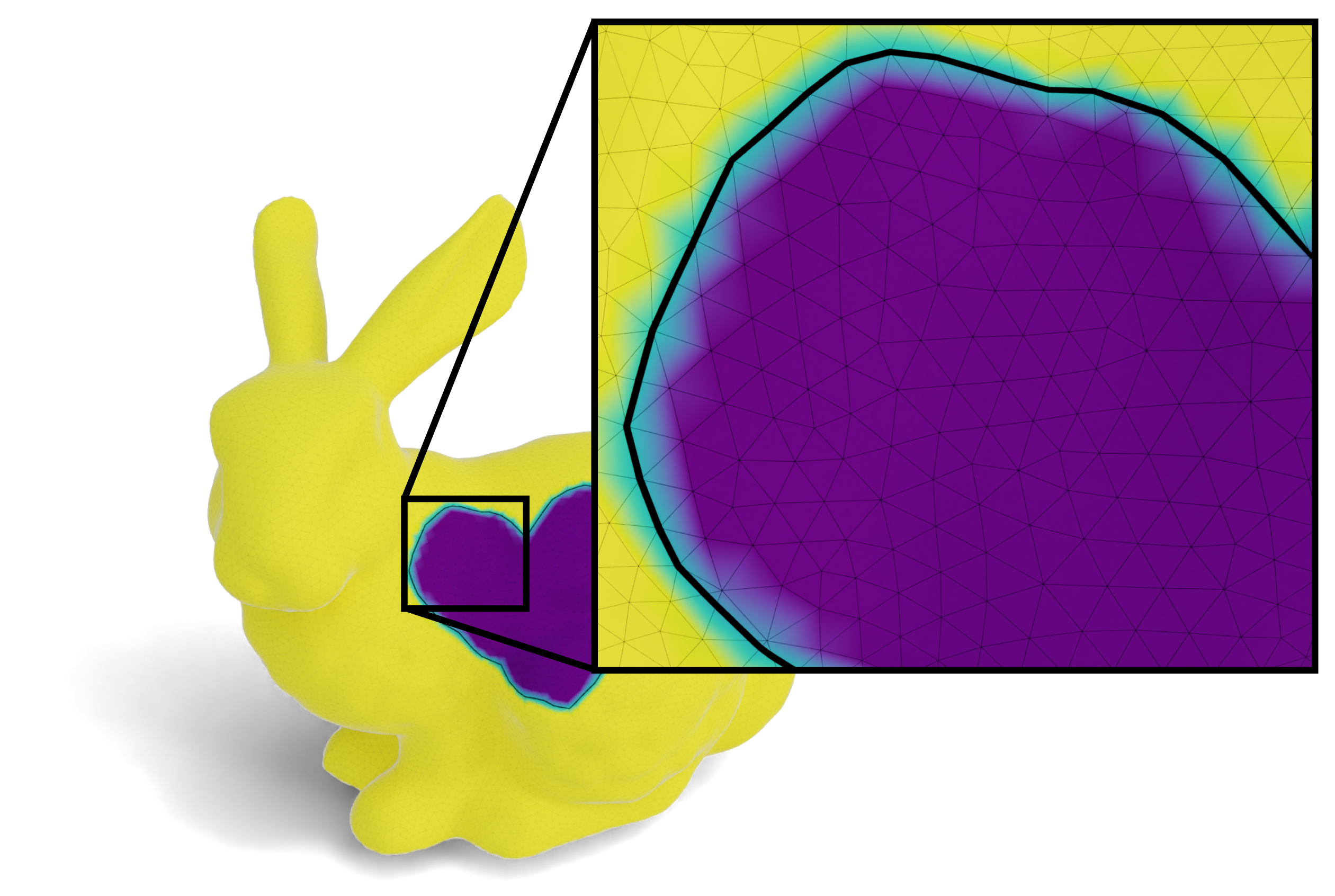We can still take the derivative of this function in the same sense of our original algorithm, and Hodge-decompose. On the other hand, integration is less clean.

## A dual formulation

A curve can be viewed as the boundary of "weighted regions", where the weights are the winding numbers. Discretely, this means if we view the curve as a 1-chain $$c$$ in a triangulation of the domain, then the winding number is a 2-chain $$b$$ such that $$\partial b=c$$.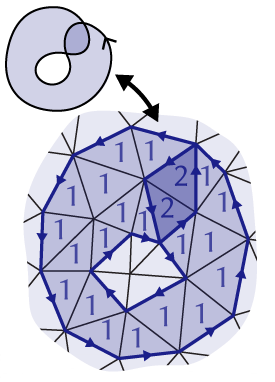This leads to a "dualized" formulation of the algorithm presented in our paper, where we solve for a dual 0-form (values per face) instead of a primal 0-form. If we encode the curve Γ as a dual 1-chain, then the relationship "Γ bounds a region $$b$$" can be expressed as the linear relation $db = c$

where $$d$$ is the discrete exterior derivative acting on dual 0-forms, and the jump condition induced by Γ is interpreted as a dual 1-form $$c$$.

The least-squares solution is given by another Laplace equation, $d\ast db = d\ast c.$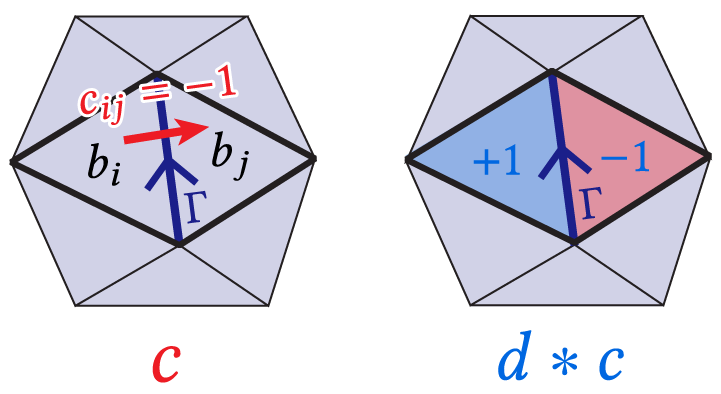To identify the nonbounding components of the curve, one can do Hodge decomposition on the derivative, just as before (although the corresponding equations will also be appropriately "dualized").

The dual formulation streamlines certain concepts; for example, the "jump derivative" we define in Section 2.4 simply becomes the usual boundary operator (we merely hint at this in Section 3.6.) Encoding the input curve as a dual 1-chain would have also subsumed more difficult situations on non-manifold and non-orientable meshes where primal 1-chains can't unambiguously specify the "sides" of the curve (see discussion in Section 2.2 and 3.7).

The dual algorithm is pretty much the same as the one presented in the paper since it solves the same linear systems and linear program, albeit on dual elements. On the other hand, the primal formulation will perhaps be more familiar to graphics practioners. For example, in the primal formulation, discretizing the jump Laplace equation yields the ordinary cotan-Laplace matrix on vertices, whereas in the dual formulation one would build an analogous cotan-Laplace matrix acting on 2-forms (though the latter isn't any more difficult to build than the former!) You also get better resolution by solving on corners (vertices), rather than on faces.

Conclusion: One approach wasn't clearly better over the other, so we picked the more familiar primal formulation.